### Home > A2C > Chapter 7 > Lesson 7.1.2 > Problem7-21

7-21.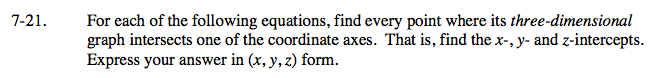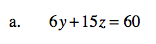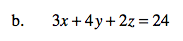To find the intercept of a given axis, set all other variables to zero.
For example, the y-intercept will be (0, ?, 0).
To find the y-intercept, let x = 0 and z = 0

Repeat the process to find the z-intercept. (0, 0, ?).

(0, 10, 0) and (0, 0, 4)

See part (a):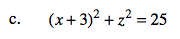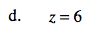See part (a).
Note: You will have two x-intercepts and two z-intercepts. Why?

See part (a):

Use the eTool below to test your solutions to parts (a), (b) and (c) above.
Click the link at right for the full version of the eTool: A2C 7-21 HW eTool